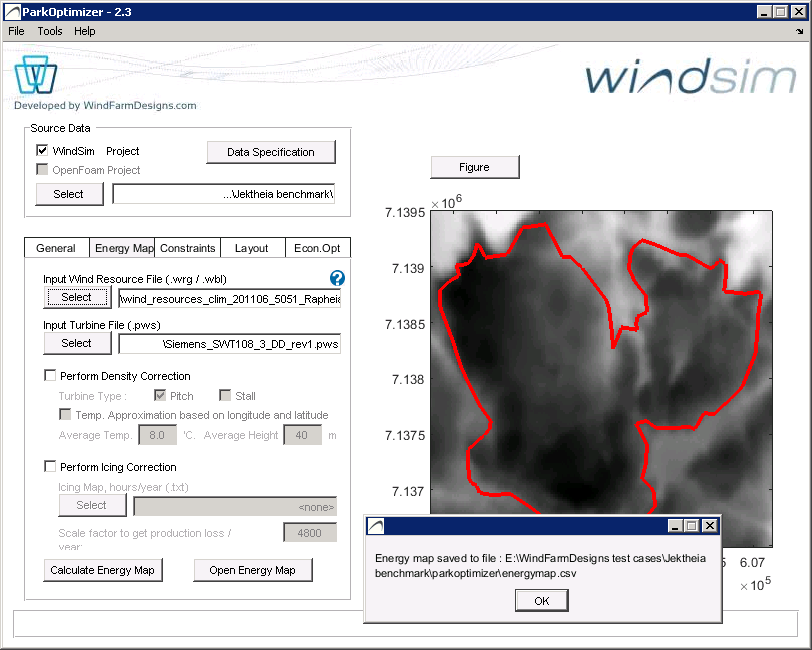# 5. Energy MapEnergy Map

## 5.1 Selecting wind- and turbine data

Select the Wind Resource wrg file, specifying the Weibull parameters for the sectors at each point, and the Turbine Specification pws file giving the power-curve PC.

The energy at each point is calculated as the sum over  sectors of

integral( wbl(v, A, k) * PC(v) * f dv ), over v=4,…,25,

where wbl is the Weibull probability distribution function with parameters (for each sector) A,k and frequency f.

After the calculation is complete energymap.csv and Google Earth energymap_ge.kml/windmap_ge.kml are saved to the Results Directory, where the windmap_ge.kml file gives average windspeed as specified in the Wind Resource file. Note that the kml files reference png bitmap files with the same name, so that the kml files must be edited if the kml/png files are moved to another location.

The energymap.csv can be loaded instead of recalculating the energy.

## 5.2 Perform density correction

Air density at site locations may vary from the standards air density = 1.225 kg/m3 conditions specified for power curves.

Two methods are provided for density correction of the Energy Map

• User  manually input average Temperature (Deg) and Height (m) above sea level of the wind farm (user input)
• Automatically correct density based on lat lon coordinates

## 5.3 Perform icing correction

Production loss from icing can be corrected for using an icing map.  The icing map can be loaded as a .txt file of coordinates and hours_icing/year .

x1 y1 hours1

x2 y2 hours2

x1 y1 hours3

..etc